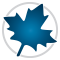MATLAB® Connectivity - Maple Features - Maplesoft# MATLAB® Connectivity

Maple provides several different connectivity options for MATLAB®.

Two-Way Integration between Maple and MATLAB®
Maple offers a technical computing solution that is tightly integrated with MATLAB®, providing direct access to all the commands, variables, and functions of each product while working in either environment.

• Enhanced integration with MATLAB® provides direct access to all of the commands, variables, and functions of each product while working in either environment.
• Access Maple's world-leading symbolic engine to handle the symbolic portions of your MATLAB® calculations and programs.
• If you are working in MATLAB®, you can still access Maple interactive assistants and tutors for rapid solution development.
• Gain arbitrary precision in your MATLAB® calculations, avoiding catastrophic cancellations in your MATLAB® code.
• Fully compatible with code written using earlier versions of symbolic toolboxes from The MathWorks™.

Flexible Connectivity Tools

MATLAB® to Maple code translation

The MATLAB® to Maple code translator helps you to convert your existing MATLAB® code into Maple for use in new or expanded projects. It also offers quick on-the-fly translations if you are more familiar with MATLAB® syntax.

• Converts and automatically executes MATLAB® commands by using the Maple equivalents
• Works with single commands or MATLAB® .m files
• Supports basic operations, matrix indexing, and matrix construction
• Over 100 MATLAB® commands are automatically mapped to their Maple equivalents
• Collection of translatable commands is user-expandable

MATLAB® Code Generation
Maple’s code generation feature can generate MATLAB® code from Maple expressions and procedures.

The MATLAB® link lets you call on MATLAB® to perform calculations from the Maple environment, and return the results to Maple for further analysis.

• Call out to MATLAB® to compute and retrieve the results of arbitrary MATLAB® expressions using flexible communication tools.
• Includes the ability to set and retrieve MATLAB® variable values from Maple.
• Any MATLAB® functions can be called, including your custom routines.
• The retrieved MATLAB® result is automatically converted to a Maple data structure.
• Directly access over a dozen common MATLAB® functions.
• Maple commands seamlessly accept both MATLAB® and Maple data structures, and call MATLAB® behind the scenes to perform the calculation.
• Direct-access functionality is available for calculating Cholesky factorizations, determinants, dimensions, eigenvalues, LU decompositions, discrete Fourier transforms, and more.

MATLAB is a registered trademark of The MathWorks Inc.

## Prêt(e) pour l'étape suivante ?

*Ce programme d'évaluation n'est proposé actuellement ni aux étudiants ni aux particuliers.

Achetez: